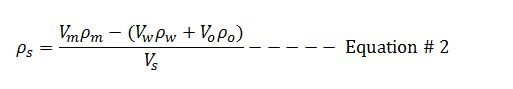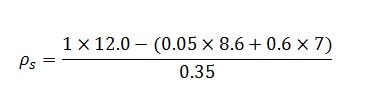# Solid Density From Retort Analysis Mud Calculation

Retort analysis is the method to determine solid and liquid components in the drilling fluid. In this article, we will adapt mass balance and retort analysis data to determine solid density in the mud.Mass balance for mud is listed below;We can rearrange the Euqation#1 in order to determine the solid densityIn the report analysis, the volume is presented in percentage and summation of solid and liquid fraction equals to one.

The unit of each parameter is described below;

Vm = mud volume, %

ρm = mud density, ppg

Vw = water volume, %

ρw = water density, ppg

Vo = oil volume, %

ρo = oil density, ppg

Vs = solid volume, %

ρs = solid density, ppg

Note: this is not only cutting weight but it includes all weights of solid (cutting and weighting material).

Example: Mud weight used for the report is 12.0 ppg and the result from the analysis showing in the following percentage;

Base oil = 60%

Solid = 35 %

Water = 5%

Base oil weight = 7.0 ppg

Water weight = 8.6 ppgρs = 21.06 ppg

Total density of solid in the drilling mud is 21.06 ppg.

### Related Post

Buoyancy Factor with Two Different Fluid Weights i... Buoyancy Factor is the factor that is used to compensate loss of weight due to immersion in drilling fluid and you can find more information from this...
Pipe Displacement Calculation Pipe displacement, normally in bbl/ft, is steel volume to displace fluid volume.  When we either pull out of hole or trip in hole for any kind of pipe...
Effect of Frictional Pressure on ECD while Forward... You may now already that the equivalent circulating density is a combination between current mud weight and frictional pressure. However, this post I ...
Drilling Formula Calculation Sheet Version 1 This is drilling formula calculation sheet v1 distributed on 28-Mar-10. I add many essential drilling formulas in to one Excel spread sheet and I woul...
Share the joy
• 23
•
•
•
•
•
•
•
•
23
Shares